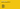# Digital and Analog Pins

Learn how to use digital and analog Pins with Micropython

In this chapter we will learn about managing digital and analog pins.

All the compatibles boards have a series of pins, most of these pins work as a general-purpose input/output (GPIO) pin. There are Digital Pins and Analog Pins depending by the signal. We will learn how to use the inputs and outputs.

There are essentially two types of pins, analog and digital pins. Digital pins can be set to either HIGH (usually 5V or 3.3V) or LOW (0V). You can use that to e.g. read a button state or to toggle an LED.

Important: unfortunately, the MicroPython implementation does not match the regular pinout of your board. This means, that if you want to use for example, digital pin (5), it might be digital pin (27) on one board, or digital pin (14) on another. Please visit the Board API article to see what the pin map for your board is.

## Digital Pins

Digital signals have two distinct values: HIGH (1) or LOW (0). You use digital signals in situations where the input or output will have one of those two values. For example you can use a digital signal to turn an LED on or off.

### Digital Write

In this section we will introduce the

``machine``
module to control the state of a pin. In this example, we will name the pin
``myLED``
.

In MicroPython we can declare a

``Pin``
with two arguments: Pin number, such as
``25``
, which defines the number of the pin that you would like to control, and
``Pin.OUT``
, to declare a pin as output.

Finally, to turn set the pin to a high or low state, we set the

``value``
to either
``1``
or
``0``
.

``````1from machine import Pin #import pin function 2
3myLED = Pin(25, Pin.OUT) #declare pin 25 as an output4
5myLED.value(1) #set pin to a high state (1) / ON6myLED.value(0) #set pin to a low state (0) / OFF``````

To create the classic "blink" example, we can also import the

``time``
module, and create a
``while``
loop.

The following examples blinks the onboard LED every second.

``````1from machine import Pin2import time3
4myLED = Pin(25, Pin.OUT) #Nano RP2040 Connect5#myLED = Pin(10, Pin.OUT) #Nano 33 BLE / Nano 33 BLE Sense6#myLED = Pin(2, Pin.OUT) #Portenta H77
8
9while True:10    myLED.value(0)11    time.sleep(1)  12    myLED.value(1)13    time.sleep(1)``````

In this example we read a digital value from a digital pin, using the

``PULL_UP``
mode. Here, we declare the pin an input through the
``Pin.IN``
command.

Then, we use

``p2.value()``
to read the state, which returns either 0 (low) or 1 (high).

``````1from machine import Pin2import utime3
4p2 = Pin(25, Pin.IN, Pin.PULL_UP)5
6while True:7    print(p2.value())8    utime.sleep(1)``````

In this example we read a digital value from a digital pin, using the

``PULL_DOWN``
mode. Here, we declare the pin an input through the
``Pin.IN``
command.

Then, we use

``p2.value()``
to read the state, which returns either 0 (low) or 1 (high).

``````1from machine import Pin2import utime3
4p2 = Pin(25, Pin.IN, Pin.PULL_DOWN)5
6while True:7    print(p2.value())8    utime.sleep(1)``````

## Analog Pins

An example of analog pin is the ADC class, that supplies an interface to analog-to-digital converters, and figures a single endpoint that can sample a continuous voltage and convert it to a discretised value.

There are four methods to use inside the ADC class:

``ADC.init``
,
``ADC.block()``
,
``ADC.read_16()``
and
``ADC.read_uv()``
.

To read an analog pin, we can use the

``ADC.read_u16``
command. This reads the specified analog pin, and return an integer in the range 0 - 65535. For this, we need to import
``ADC``
and
``Pin``
from the
``machine``
module.

``````1import machine2import time3
4# Make sure to follow the GPIO map for the board you are using.5# Pin 29 in this case is the "A3" pin on the Nano 33 BLE / BLE Sense6adc_pin = machine.Pin(29) 7adc = machine.ADC(adc_pin)8

## PWM (Pulse Width Modulation)

PWM is used to produce analog results with digital means, by switching ON/OFF a signal rapidly.

As a result, you can simulate a specific voltage written to a pin. In the example below, we write

``30000``
in a range between 0 - 65535 (16 bits), which if you connect an LED to the pin, it will be on at about "half" capacity.

For this, we need to import

``PWM``
and
``Pin``
from the
``machine``
module.

``````1from machine import Pin, PWM, ADC2
3pwm = PWM(Pin(15))4duty = 30000 #between 0-650005
6pwm.freq(1000)7
8while True:9    pwm.duty_u16(duty)``````